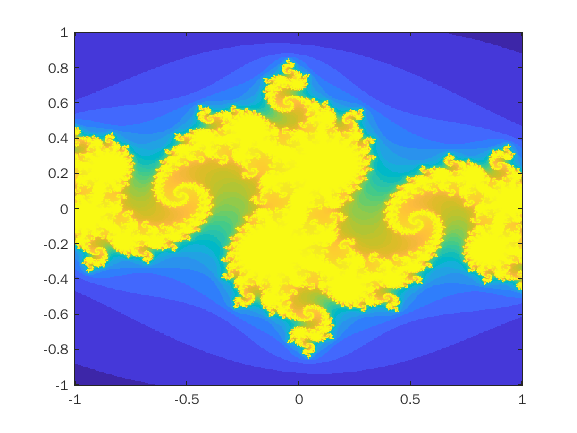# Julia Set AnimationBarath Narayanan on 5 Oct 2021
• 57
• 327
• 16
• 0
• 259
Bound = 1;
C = -0.8+1i*0.156;
N = 100;
temp = linspace(-Bound, Bound, 500);
R = repmat(temp,500,1);
I = transpose(R);
Z = R + (1i*I);
M = repmat(N,500,500);
for n = (1 : N)
Z = Z.^2 + C;
M((abs(Z) > 2)) = n;
Z((abs(Z) > 2)) = NaN;
imagesc(temp,temp,atan(0.1*M)); axis xy; drawnow;
end